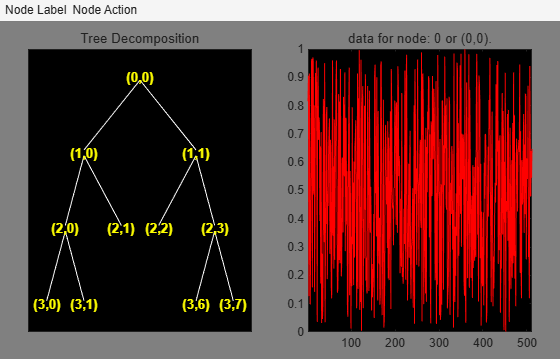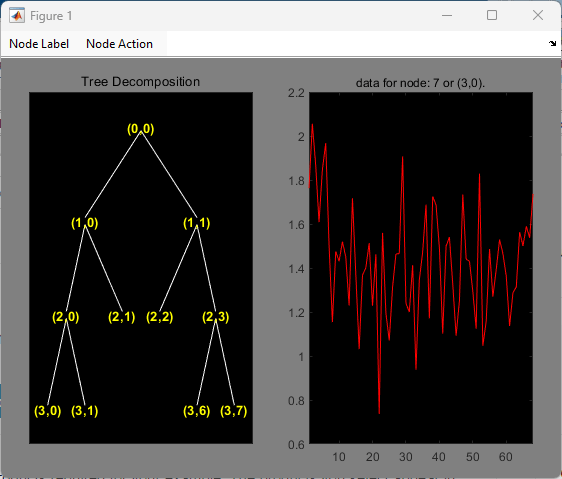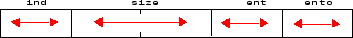# wptree

WPTREE constructor

## Syntax

``T = wptree(order,depth,x,wname)``
``T = wptree(order,depth,x,wname,enttype,entpar)``
``T = wptree(order,depth,x,wname,enttype,entpar,userdata)``

## Description

example

````T = wptree(order,depth,x,wname)` returns a complete wavelet packet tree `T` of order `order` corresponding to a wavelet packet decomposition of `x` at level `depth`, using Shannon entropy and the wavelet specified by `wname`.`T = wptree(order,depth,x,wname)` is equivalent to ```T = wptree(order,depth,x,wname,'shannon')```.```
````T = wptree(order,depth,x,wname,enttype,entpar)` uses the entropy type specified by `enttype`. `entpar` is an optional parameter depending on the value of `enttype`.```
````T = wptree(order,depth,x,wname,enttype,entpar,userdata)` sets the userdata field of `T`.```

## Examples

collapse all

Create a 1-D signal.

`x = rand(1,512);`

Create the wavelet packet decomposition tree associated with the wavelet packet decomposition of the signal at level 3 using the `db3` wavelet.

`t = wptree(2,3,x,"db3");`

Recompose the fourth and fifth nodes of the tree. Plot the result.

```t = wpjoin(t,[4;5]); plot(t)```Click the node `(3,0)` to get this figure:## Input Arguments

collapse all

Order of the tree, specified as `2` or `4`. The order of the tree is the number of children of each nonterminal node. If `x` is a vector (1-D signal), specify an order of `2`. If `x` is a matrix (image), specify an order of `4`.

Data Types: `double`

Level of wavelet packet decomposition, specified as a positive integer.

Data Types: `double`

Input data, specified as a vector (signal) or matrix (image).

Data Types: `double`

Wavelet name, specified as a character vector or string scalar. For more information, see `wfilters`.

Data Types: `string` | `char`

Entropy type, specified as a character vector or string scalar. For more information, see `wpdec` or `wpdec2`

Data Types: `string` | `char`

Optional parameter used for entropy computation. For more information, see `wpdec` or `wpdec2`.

Data Types: `double` | `string` | `char`

User data to set in the `userdata` field of `T`, specified as an array, cell array, or structure array.

Example: ```t = wptree(2,3,x,'db3','sure',0.5,{1,"aa",rand(3,3)})```

## Output Arguments

collapse all

Wavelet packet tree, returned as a `WPTREE` object.

• If `order = 2`, `T` is a `WPTREE` object corresponding to a wavelet packet decomposition of the vector (signal) `x`, at level `depth` with a particular wavelet `wname`.

• If `order = 4`, `T` is a `WPTREE` object corresponding to a wavelet packet decomposition of the matrix (image) `x`, at level `depth` with a particular wavelet `wname`.

The `WPTREE` object has these fields:

 `'dtree'` DTREE parent object `'wavInfo'` Structure (wavelet information) `'entInfo'` Structure (entropy information)

For more information on object fields, see the `get` function or type

```help wptree/get ```

The wavelet information structure, `'wavInfo'`, contains

 `'wavName'` Wavelet name `'Lo_D'` Low Decomposition filter `'Hi_D'` High Decomposition filter `'Lo_R'` Low Reconstruction filter `'Hi_R'` High Reconstruction filter

The entropy information structure, `'entInfo'`, contains

 `'entName'` Entropy name `'entPar'` Entropy parameter

Fields from the DTREE parent object:

 'allNI' All nodes information

`'allNI'` is an array of size `nbnode` by `5`, which contains

 `ind` Index `size` Size of data `ent` Entropy `ento` Optimal entropy

Each line is built based on this scheme:## Version History

Introduced before R2006a